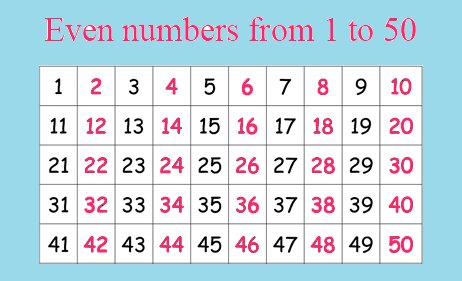# Even Numbers 1 to 100 in C || Print 1 to 50 Even NumbersPrint Even Number

## In this program, you will learn how to print 1 to 100 even numbers and 1 to 50 even numbers just follow the program. Even numbers mean the number which is divisible by 2.

### Example #1: Even Numbers 1 to 100

#include<stdio.h>

main()

{

int i=1;

for(i=1;i<=100;i=i+1)

{

if(i%2==0)

printf("%d\t",i);

}

getch();

}

### Output of Program:Print 1-100 Even Numbers

### Example #2: Even Numbers 1 to 50

#include<stdio.h>

main()

{

int i=1;

for(i=1;i<=50;i=i+1)

{

if(i%2==0)

printf("%d\t",i);

}

getch();

}

### Output of Program:Print 1 to 50 even numbers